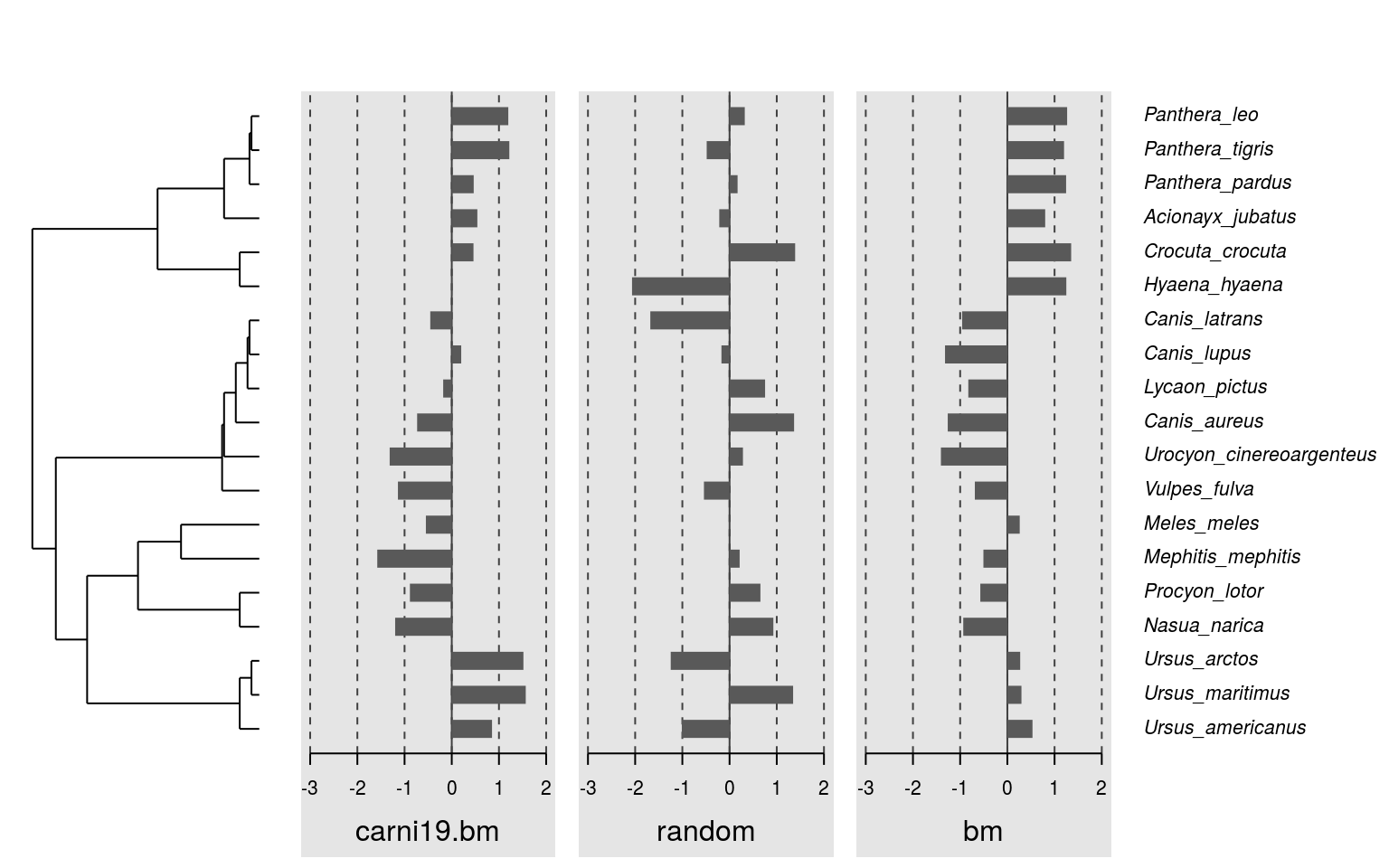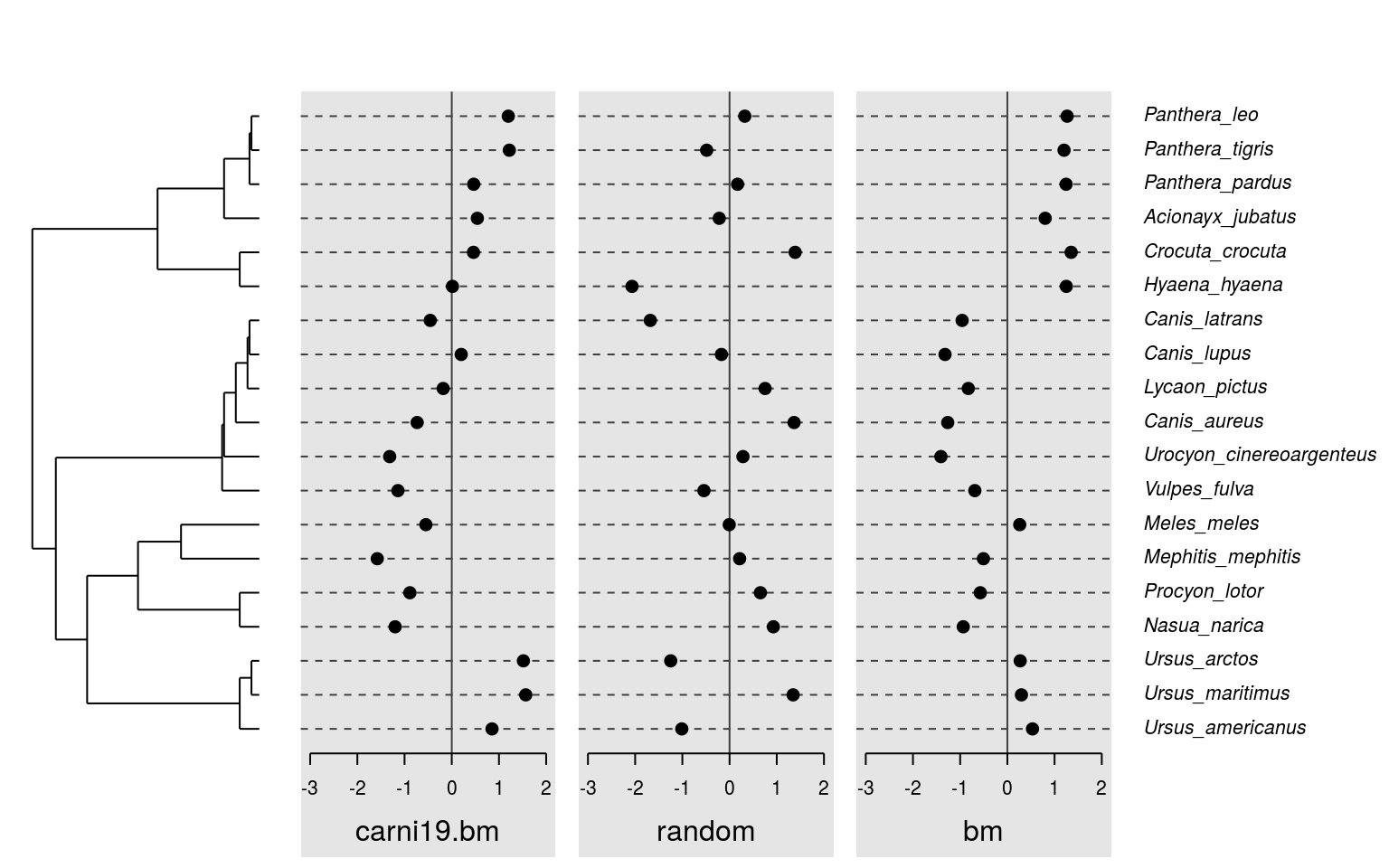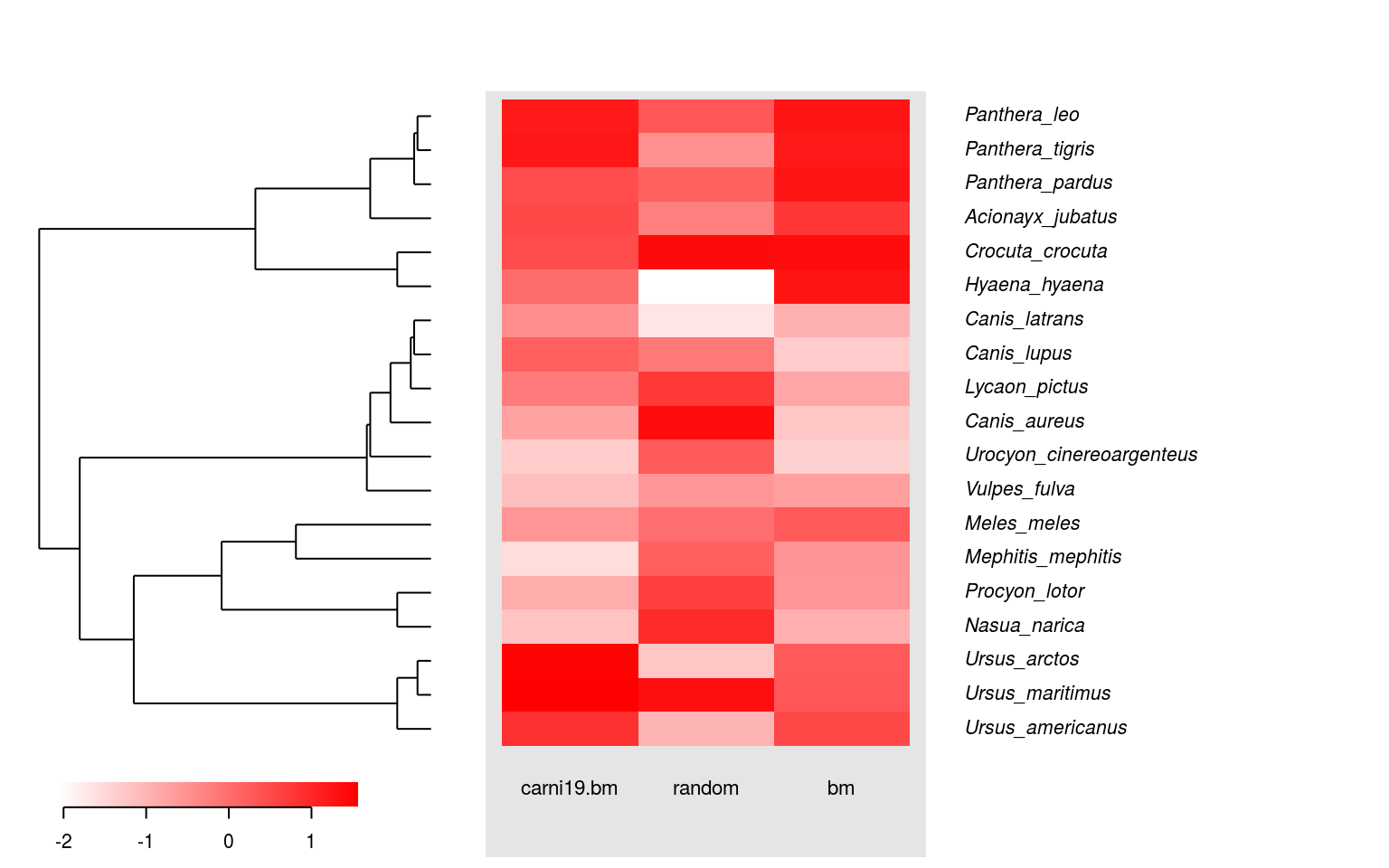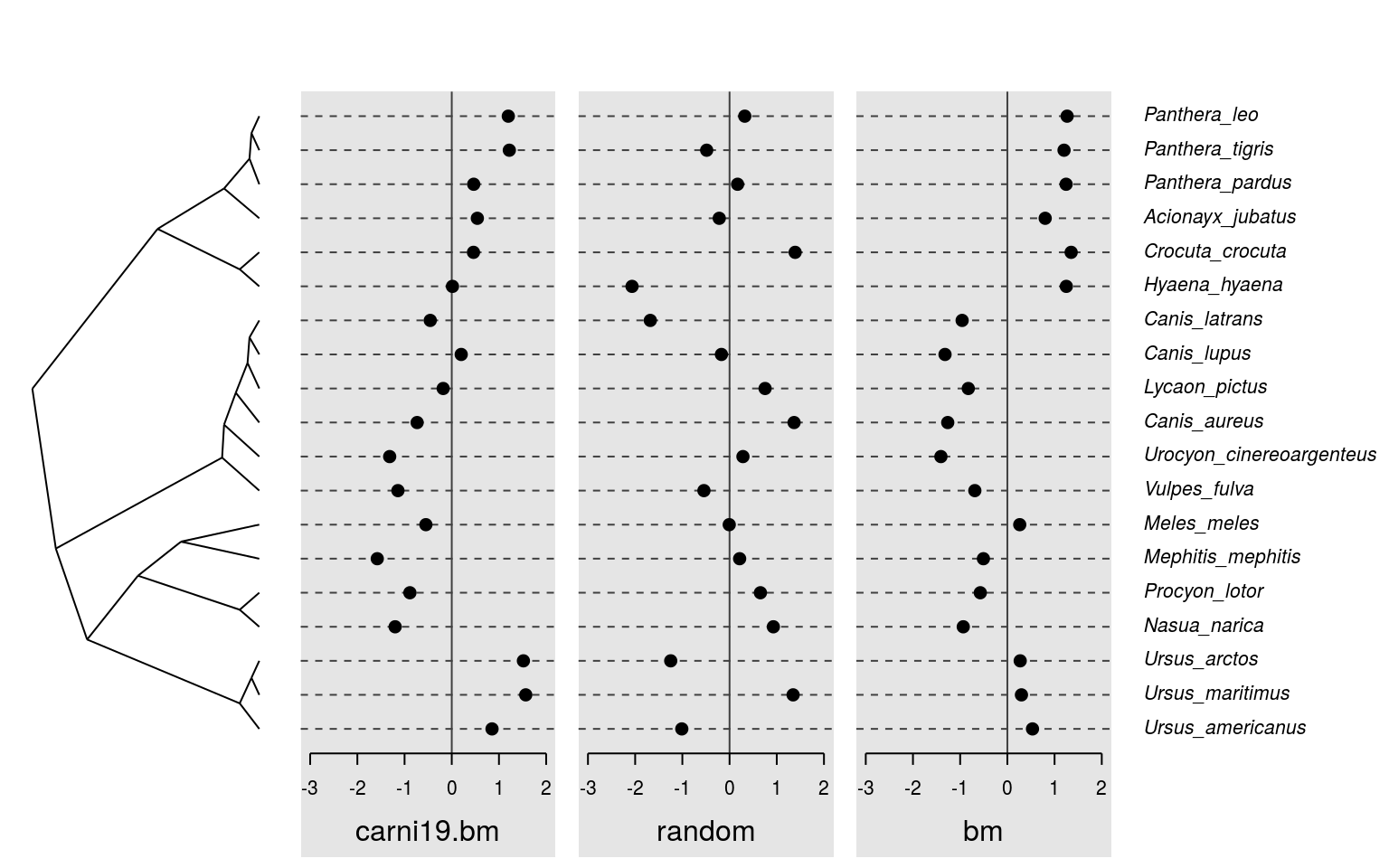# Introducing the plotting capabilities of the `phylosignal` package

The `phylosignal` package comes with functions designed to plot trait values and phylogeny together. These functions are generics for `phylo4d` objects. This document present how to use them.

## Data

First, we load the package `phylosignal` and some others. We will also use the dataset `carnivora` from `adephylo`.

``````library(ape)
library(phylobase)
library(phylosignal)
data(carni19)``````

Here is a phylogenetic tree of 19 carnivora species.

``tre <- read.tree(text = carni19\$tre)``

And we create a dataframe of 3 traits for the 19 carnivora species. - Body mass - Random values - Simulated values under a Brownian Motion model along the tree

``````dat <- data.frame(carni19\$bm)
dat\$random <- rnorm(dim(dat), sd = 10)
dat\$bm <- rTraitCont(tre)``````

We can combine phylogeny and traits into a `phylo4d` object.

``p4d <- phylo4d(tre, dat)``

## Basics

Once we have a `phylo4d` object, we can plot it… There are three plotting functions: `barplot`, `dotplot` and `gridplot`. These functions are actually wrappers of the function `multiplot.phylo4d`.

``barplot(p4d)````dotplot(p4d)````gridplot(p4d)``Each of these functions can be used with one of the three tree styles: `phylogram`, `cladogram` and `fan`. For example, here is a dotplot with a cladogram.

``dotplot(p4d, tree.type = "cladogram")``And here a gridplot with a fan tree.

``gridplot(p4d, tree.type = "fan", tip.cex = 0.6, show.trait = FALSE)``